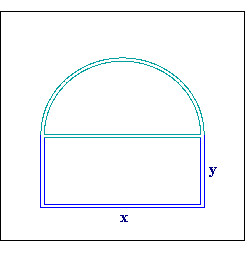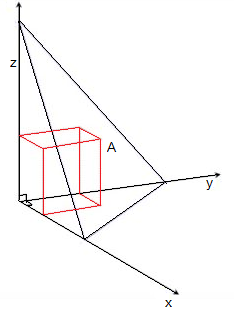# Optimization Problems in Calculus

Contents:

More optimization problems:

## General Optimization StepsOptimization problems in calculus often involve the determination of the “optimal” (meaning, the best) value of a quantity. For example, we might want to know:

• The biggest area that a piece of rope could be tied around.
• How high a ball could go before it falls back to the ground.
• At which point of a loop does a roller coaster run the slowest.

Very often, the optimization must be done with certain constraints. In the case of the rope, we’re limited by its length. These constraints are usually very helpful to solve optimization problems (for an advanced example of using constraints, see: Lagrange Multiplier). Optimal values are often either the maximum or the minimum values of a certain function.

## Optimization Problems in Calculus: Steps.

Example problem: Find the maximum area of a rectangle whose perimeter is 100 meters. (Note: This is a typical optimization problem in AP calculus).

Step 1: Determine the function that you need to optimize. In the example problem, we need to optimize the area A of a rectangle, which is the product of its length L and width W. Our function in this example is:
A = LW.

Step 2: Identify the constraints to the optimization problem. In our example problem, the perimeter of the rectangle must be 100 meters. This will be useful in the next step.

Step 3: Express that function in terms of a single variable upon which it depends, using algebra. For this example, we’re going to express the function in a single variable. “L.”

1. A rectangle’s perimeter is the sum of its sides, that is, 100m = 2L + 2W.
2. Subtract 2L from both sides of this equation, 2W = 100m – 2L.
3. Divide each side by 2: W = 50m – L.
4. Substitute 50m – L for “W” in A = LW: A = L (50m – L) = 50m L – L².

Step 4: Calculate the derivative of the function with respect to a variable.

• dA/dL = 50m (1) L(1 – 1) – 2 L(2-1) = 50m – 2L.

Step 5: Set the function to zero and compute the corresponding variable’s value. For our sample problem, we set:

• dA/dL = 0 = 50m – 2L.
• So, L = 25m.

Step 6: Use the value from Step 5 to calculate the corresponding optimal value of the function. In our sample problem, A = 50m L – L2 = 50 m (25m) – (25m)2 = 625 m2.

## The Volume of the Largest Rectangular Box Inscribed in a Pyramid

If you’re asked to find the volume of the largest rectangular box in the first octant, with three faces in the coordinate planes and one vertex in a given plane, you’re being asked to find the volume of the largest rectangular box that fits in a pyramid like the one below.Imagine that point A is embedded in the rectangular face.

Suppose the plane is x + 2y + 3z = 6. What is the volume of the largest box?

Your first step should be to define the volume. Since your box is rectangular, the formula is:
width x depth x height.

We can write this as:

V = xyz

Since x + 2y + 3z = 6, we know z = (6 – x – 2y) / 3. We can substitute that in our volume equation to create a function that tells us the volume in terms of x and y:

V = f(x, y) = xy(6 – x – 2y) / 3

We’re looking for a maximum, so we want to find the point where the derivative of volume with respect to both x and y is zero.

We can call those two derivatives fx and fy, and we can calculate them as

• fx = ⅓y (6 – 2x – 2y) = 0 → y = 0, y = 3 – x
• fy = ⅓x (6 – x – 4y) = 0

At y = 0, equation B becomes (1/3)x (6 – x) = 0, so x = 0 or x = 6.

At y = 3 – x, equation B becomes x(-2 + x) = 0, so the zeros are x = 0 (in which case y = 3) or x = 2 (in which case y = 1).

We’e just found the four critical points: (6, 0), (0,0) (0, 3), (2, 1).

We can draw each of these points on a 3D graph, and use our knowledge of geometry to decide which box is the maximum: it should be fairly evident that it will be (2,1), and since x = 2 and y = 1 leads to z = 2/3, the coordinate of the largest box will be (2, 1, 2/3) .

## Alternative: Second Derivative Test

If you don’t want to rely on geometry, you can use the second derivative test to find out which critical point gives us that box with maximum volume:

D = D(a, b) = fxx(a,b) fyy(a, b) – [fxy(a, b)]2at (6, 0), (0, 0), (0, 3)
D = 0 – 4 = -4
at (2, 1)Which leads to the same conclusion.

So the volume V = xyz = 2 · 1 · 2/3 = 4/3.

## Optimization Problems: References

Miech, Ron. Calculus Problems: Volume of the Largest Rectangular Box. Retrieved from http://www.math.ucla.edu/~ronmiech/Calculus_Problems/32A/chap12/section7/820d45/820_45.html on January 1, 2019.
Formula for the volume of a rectangular box.

CITE THIS AS:
Stephanie Glen. "Optimization Problems in Calculus" From StatisticsHowTo.com: Elementary Statistics for the rest of us! https://www.statisticshowto.com/calculus-problem-solving/optimization-problems/